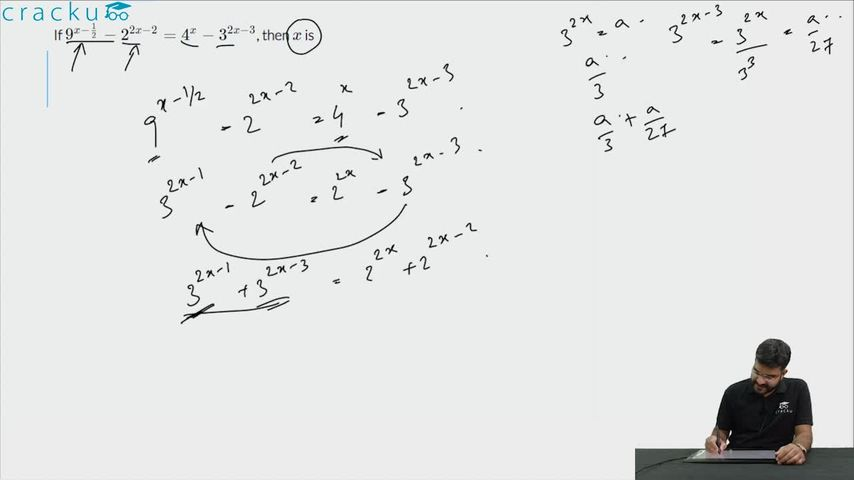Question 91

# If $$9^{x-\frac{1}{2}}-2^{2x-2}=4^{x}-3^{2x-3}$$, then $$x$$ is

Solution

It is given that $$9^{x-\frac{1}{2}}-2^{2x-2}=4^{x}-3^{2x-3}$$

Let us try to reduce them to powers of $$3$$ and $$2$$
The given equation can be reduced to $$3^{2x-1} + 3^{2x-3} = 2^{2x} + 2^{2x-2}$$

Hence, $$3^{2x-3} \times 10 = 2^{2x-2} \times 5$$
Therefore, $$3^{2x-3} = 2^{2x-3}$$

This is possible only if $$2x-3=0$$ or $$x=3/2$$

### View Video Solution